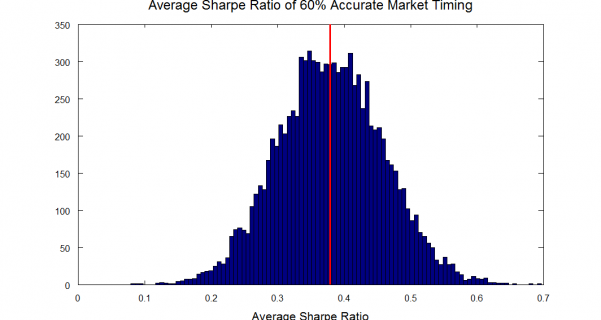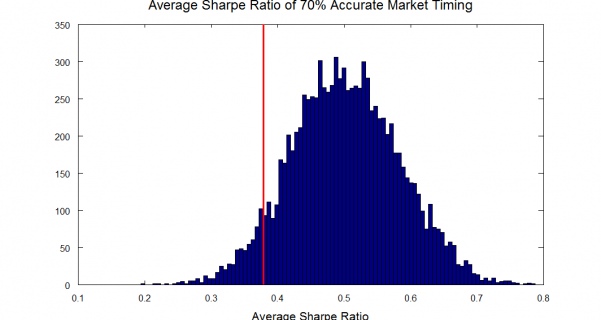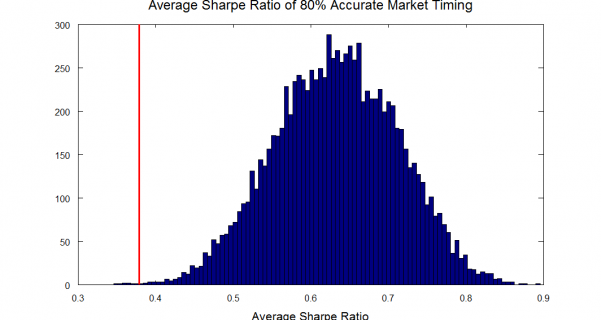# Market Timing: How good is good enough?

Feb 212011

Monte Carlo Simulations using return data from 1926-2010

In 1975, Nobel laureate William Sharpe published a study titled “Likely Gains from Market Timing”. In this paper, Sharpe reportedly found that a market timer who switches between 100% stocks and 100% T-bills on an annual basis must be correct about 74% of the time (on average) to beat the market.

Unfortunately, a free copy of this paper is not available on the web, and I don’t have access to this much cited paper. However, I’ve seen a number of related analyses based on Sharpe’s approach, and I believe we can recreate a similar result through simulation.

In this post, I’ll simulate a simple market timing strategy and determine the market timing accuracy required to outperform buy-and-hold. I’ll compare market timing to buy-and-hold in terms of both total returns and risk-adjusted returns (measured by the Sharpe Ratio). I’m going to use market and T-bill returns for the years 1927-2010.

My assumptions are that an investor makes a decision at the beginning of each year to invest in either stocks or T-bills, based on his/her prediction for the coming year. The Monte Carlo simulation is run using the actual annual returns for stocks and T-bills from 1927 to 2010, and 10,000 runs of the simulation are plotted to generate a distribution.

The Monte-Carlo results for 50% prediction accuracy are shown below.  The red line represents the arithmetic average return for a buy and hold investor over the years from 1926 thru 2010, and the distribution represents the market timing outcomes for the 10,000 trials:Clearly, the investor who decides between stocks and T-bills with only 50 percent accuracy has a very high chance of underperforming the market.  The results do look slightly better if we compare the potential Sharpe ratios (i.e. the risk adjusted return) generated by this strategy to the Sharpe ratio realized by the buy and hold investor.On average, the 50% accurate investor still tends to underperform after adjusting for risk, but there is now at least a small chance of outperforming buy-and-hold.  This occurs because the investor with 50% accurate timing spends a lot of time invested in risk-free assets (T-bills).

If we repeat these tests with 60% accurate timing we get the following:We can see that, with 60% accurate market timing, there is still a high chance of underperforming buy-and-hold.  However, on a risk adjusted basis, the average results are similar to buy-and-hold.

If we examine the 1926-2010 return data closely, we find that the stocks outperform T-bills about 67% of the time, so the buy and hold investor who holds only stocks is actually invested in the higher performing asset class about 67% of the time.  For this reason, we might expect that as accuracy goes to 70% we will see the average returns of the market timer outperform the buy and hold.

Here are the results with 70% accuracy:These simulation results show that the average returns of a market timer with 70% accuracy still underperform the buy and hold investor slightly over half the time.   This is true even though the buy and hold investor is correct only about 67% of the time.   The reason for this result is that the gain in return, when the investor correctly picks T-bills over stocks, is less, on average, than the loss of return when the market timer incorrectly picks T-bills over stocks.

Here are the results for 80% accurate market timing:With 80% accuracy we finally see the market timer outperform the returns of the buy-and-hold investor.  However, note that, on average, the return is less than 2% higher.  Therefore, if the market-timer is paying an advisor 2% of assets for timing advice, then even 80% timing will not be good enough!

Conclusion:

This analysis illustrates the difficulties faced by an aspiring market timer.  A very high accuracy is required to beat the buy and hold investor in terms of raw returns, and an even higher accuracy is required if we consider additional costs such as management fees or trading costs.  Our simulation results support Sharpe’s conclusion that the minimum accuracy required is over 70%.

On a risk adjusted basis, the results look better, but we still need an accuracy of over 60% for market timing to pay off.

Supplemental Information:

Data:

The data for this analysis is from the Kenneth French website.  The annual returns were extracted from the Fama-French Factor Data, and stored in a file named “F-F_Factors_annual.txt”.

Code:

The Octave code used to generate these plots is shown here:

```clear all;
close all;

% Extract Data Columns
annual_stock_dates = ff_returns(:,1);
annual_stock_return = ff_returns(:,2)/100 + ff_returns(:,5)/100;
annual_tbill_return = ff_returns(:,5)/100;

%plot(annual_stock_return);
%figure
%plot(annual_tbill_return);

%% Part 1 Calculations

% Stock beating tbills
percent_stocks_exceeds_tbills = 100* sum((annual_stock_return - annual_tbill_return)>0)/length(annual_stock_return);

% 10,000 Random Guesses

returns = [annual_stock_return annual_tbill_return];
for i = 1:10000
% Guess t-bill or stock with 50/50 probability
guess = 1+round(rand(length(annual_stock_return),1));
for j = 1:length(annual_stock_return)
return_draw(j) = returns(j,guess(j));
end
wealth(i) = prod(1+return_draw);
averagereturn(i) = mean(return_draw);
stdreturn(i) = std(return_draw);
sharpe(i) = mean(return_draw - annual_tbill_return')/std(return_draw - annual_tbill_return');
end

%% Imperfect Timing

returns = [annual_stock_return annual_tbill_return];

% Generate Random values for all sims
guess = rand(length(annual_stock_return),10000);

%%%%%%%%%%%%%%%%%%%%%%%%%%%%%%%%%%%%%%%%%%%%%%%%%%%%%%%%%%%%%%%%%%%%%%%%
% 50% Accuracy
%%%%%%%%%%%%%%%%%%%%%%%%%%%%%%%%%%%%%%%%%%%%%%%%%%%%%%%%%%%%%%%%%%%%%%%%
for i = 1:10000
for j = 1:length(annual_stock_return)
if guess(j,i) < 0.5
return_draw(j) =max(returns(j,:));
else
return_draw(j) = min(returns(j,:));
end
end
wealth50(i) = prod(1+return_draw);
averagereturn50(i) = mean(return_draw);
stdreturn50(i) = std(return_draw);
sharpe50(i) = mean(return_draw - annual_tbill_return')/std(return_draw - annual_tbill_return');
end

figure;
hist(averagereturn50,100)
title('Average Return of 50% Accurate Timing','fontsize',18)
xlabel('Average Return','fontsize',16)
hold on
line([mean(annual_stock_return) mean(annual_stock_return)], [ylim()], 'linewidth',3.5,'color','red')
hold off

figure;
hist(sharpe50,100)
title('Average Sharpe Ratio of 50% Accurate Market Timing','fontsize',18)
xlabel('Average Sharpe Ratio','fontsize',16)
hold on
line([mean(annual_stock_return-annual_tbill_return)/std(annual_stock_return-annual_tbill_return)...
mean(annual_stock_return-annual_tbill_return)/std(annual_stock_return-annual_tbill_return) ],...
[ylim()], 'linewidth',3.5,'color','red')
hold off

%%%%%%%%%%%%%%%%%%%%%%%%%%%%%%%%%%%%%%%%%%%%%%%%%%%%%%%%%%%%%%%%%%%%%%%%
% 60% Accuracy
%%%%%%%%%%%%%%%%%%%%%%%%%%%%%%%%%%%%%%%%%%%%%%%%%%%%%%%%%%%%%%%%%%%%%%%%
for i = 1:10000
for j = 1:length(annual_stock_return)
if guess(j,i) < 0.6
return_draw(j) =max(returns(j,:));
else
return_draw(j) = min(returns(j,:));
end
end
wealth60(i) = prod(1+return_draw);
averagereturn60(i) = mean(return_draw);
stdreturn60(i) = std(return_draw);
sharpe60(i) = mean(return_draw - annual_tbill_return')/std(return_draw - annual_tbill_return');
end

figure;
hist(averagereturn60,100)
title('Average Return of 60% Accurate Timing','fontsize',18)
xlabel('Average Return','fontsize',16)
hold on
line([mean(annual_stock_return) mean(annual_stock_return)], [ylim()], 'linewidth',3.5,'color','red')
hold off

figure;
hist(sharpe60,100)
title('Average Sharpe Ratio of 60% Accurate Market Timing','fontsize',18)
xlabel('Average Sharpe Ratio','fontsize',16)
hold on
line([mean(annual_stock_return-annual_tbill_return)/std(annual_stock_return-annual_tbill_return)...
mean(annual_stock_return-annual_tbill_return)/std(annual_stock_return-annual_tbill_return) ],...
[ylim()], 'linewidth',3.5,'color','red')
hold off

%%%%%%%%%%%%%%%%%%%%%%%%%%%%%%%%%%%%%%%%%%%%%%%%%%%%%%%%%%%%%%%%%%%%%%%%
% 70% Accuracy
%%%%%%%%%%%%%%%%%%%%%%%%%%%%%%%%%%%%%%%%%%%%%%%%%%%%%%%%%%%%%%%%%%%%%%%%
for i = 1:10000
for j = 1:length(annual_stock_return)
if guess(j,i) < 0.7
return_draw(j) =max(returns(j,:));
else
return_draw(j) = min(returns(j,:));
end
end
wealth70(i) = prod(1+return_draw);
averagereturn70(i) = mean(return_draw);
stdreturn70(i) = std(return_draw);
sharpe70(i) = mean(return_draw - annual_tbill_return')/std(return_draw - annual_tbill_return');
end

figure;
hist(averagereturn70,100)
title('Average Return of 70% Accurate Timing','fontsize',18)
xlabel('Average Return','fontsize',16)
hold on
line([mean(annual_stock_return) mean(annual_stock_return)], [ylim()], 'linewidth',3.5,'color','red')
hold off

figure;
hist(sharpe70,100)
title('Average Sharpe Ratio of 70% Accurate Market Timing','fontsize',18)
xlabel('Average Sharpe Ratio','fontsize',16)
hold on
line([mean(annual_stock_return-annual_tbill_return)/std(annual_stock_return-annual_tbill_return)...
mean(annual_stock_return-annual_tbill_return)/std(annual_stock_return-annual_tbill_return) ],...
[ylim()], 'linewidth',3.5,'color','red')
hold off

%%%%%%%%%%%%%%%%%%%%%%%%%%%%%%%%%%%%%%%%%%%%%%%%%%%%%%%%%%%%%%%%%%%%%%%%
% 80% Accuracy
%%%%%%%%%%%%%%%%%%%%%%%%%%%%%%%%%%%%%%%%%%%%%%%%%%%%%%%%%%%%%%%%%%%%%%%%
for i = 1:10000
for j = 1:length(annual_stock_return)
if guess(j,i) < 0.8
return_draw(j) =max(returns(j,:));
else
return_draw(j) = min(returns(j,:));
end
end
wealth80(i) = prod(1+return_draw);
averagereturn80(i) = mean(return_draw);
stdreturn80(i) = std(return_draw);
sharpe80(i) = mean(return_draw - annual_tbill_return')/std(return_draw - annual_tbill_return');
end

figure;
hist(averagereturn80,100)
title('Average Return of 80% Accurate Timing','fontsize',18)
xlabel('Average Return','fontsize',16)
hold on
line([mean(annual_stock_return) mean(annual_stock_return)], [ylim()], 'linewidth',3.5,'color','red')
hold off

figure;
hist(sharpe80,100)
title('Average Sharpe Ratio of 80% Accurate Market Timing','fontsize',18)
xlabel('Average Sharpe Ratio','fontsize',16)
hold on
line([mean(annual_stock_return-annual_tbill_return)/std(annual_stock_return-annual_tbill_return)...
mean(annual_stock_return-annual_tbill_return)/std(annual_stock_return-annual_tbill_return) ],...
[ylim()], 'linewidth',3.5,'color','red')
hold off
```

### 2 Responses to “Market Timing: How good is good enough?”

1. […] —which is about what you would expect from tossing a coin. In 1975, Nobel laureate William Sharpe found that a market timer who switches between 100 percent stocks and 100 percent Treasury bills annually […]

2. […] is about what you would expect from tossing a coin. In 1975, Nobel laureate William Sharpe found that a market timer who switches between 100 percent stocks and 100 percent Treasury bills annually […]